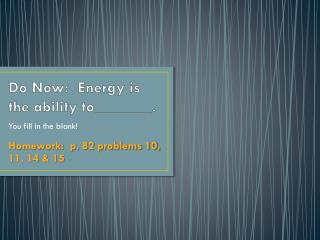Download PresentationDo Now: Energy is the ability to_______.# Do Now: Energy is the ability to_______. - PowerPoint PPT Presentation

Download Presentation##### Do Now: Energy is the ability to_______.

Download Policy: Content on the Website is provided to you AS IS for your information and personal use and may not be sold / licensed / shared on other websites without getting consent from its author. While downloading, if for some reason you are not able to download a presentation, the publisher may have deleted the file from their server.

- - - - - - - - - - - - - - - - - - - - - - - - - - - E N D - - - - - - - - - - - - - - - - - - - - - - - - - - -
##### Presentation Transcript

1. Do Now: Energy is the ability to_______. You fill in the blank! Homework: p. 82 problems 10, 11, 14 & 15

2. Chapter 3.2 Objectives & Vocabulary • Describe work and energy. • Calculate potential energy. • Calculate kinetic energy. • Apply the law of conservation of energy to explain the motion of an object acted on by gravity. • energy • joule • work • potential energy • kinetic energy • law of conservation of energy

3. What is Energy? • The ability to do work or cause change. • What is work? Work is the energy used to move something. We measure it in Joules. • Energy has a number of different forms, all of which measure the ability of an object to do work on or change another object. • In other words, there are different ways that an object can possess energy.

4. How do we define work mathematically?

5. What are the different forms of Energy? Mechanical Energy • Kinetic Energy • Potential Energy • Thermal, or heat energy • Chemical Energy • Electrical Energy • Electrochemical Energy • Electromagnetic Energy (light) • Sound Energy • Nuclear Energy

6. Potential vs. Kinetic Energy Define Potential Energy Define Kinetic Energy Kinetic energy is the energy of motion. Kinetic energy is measured by how much is work done to put an object in motion or to rest. Potential energy is energy due to position or stored energy. Potential energy is also called gravitational potential energy.

7. EXAMPLES OF POTENTIAL & KINETIC • By stretching a rubber band, you give it potential energy. A vase on a shelf has stored potential energy. A football being held by a quarterback has potential energy until it is thrown and it turns into kinetic energy. • A basketball player has kinetic energy. The movements that she does show the energy that is being displayed while she is moving. When you are running, walking, or jumping, your body is exhibiting kinetic energy.

8. Two basic types of energy!

9. Let’s look at an example… A 2 kg rock is at the edge of a cliff 20 meters above a lake. It becomes loose and falls toward the water below. Calculate its potential and kinetic energy when it is at the top and when it is halfway down. Its speed is 14 m/sec at the halfway point. You can assume the initial speed is 0 m/sec because the rock starts from rest. E p= mgh and Ek = ½ mv2 Potential energy at the top: m = 2 kg, g = 9.8 N/kg, and h = 20 mEp=(2 kg)(9.8 N/kg)(20 m) = 392 J Potential energy halfway down: m= 2 kg, g = 9.8 N/kg, and h = 10 m Ep=(2 kg)(9.8 N/kg)(10 m) = 196 J Kinetic energy at the top: m = 2 kg and v = 0 m/sec Ek=(1/2)(2 kg)(02)=0 J Kinetic energy halfway down: m= 2 kg and v = 14 m/sec Ek=(1/2)(2 kg)(14 m/sec)2 = 196 J

10. YOUR TURN… • b. Calculate the kinetic energy of a 4 kilogram cat running at 5 m/sec. • Answer: 50 J • Calculate the potential energy of a 4 kilogram cat crouched 3 meters off the ground. Answer: 117.6 J